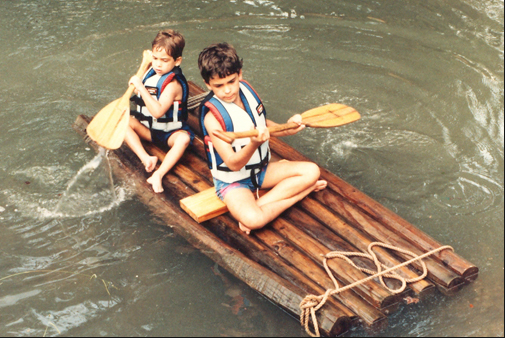Raft for childrenTwo children, each weighing $534 \text{ N,}$ make a log raft by lashing together logs. Each log has a diameter of $0.30 \text{ m}$ and a length of $1.80 \text{ m}.$ How many logs will be needed to keep them afloat in fresh water?

The density of the logs is $800 \text{ kg/m}^3$.
The density of fresh water is $1000 \text{ kg/m}^{3}.$

×